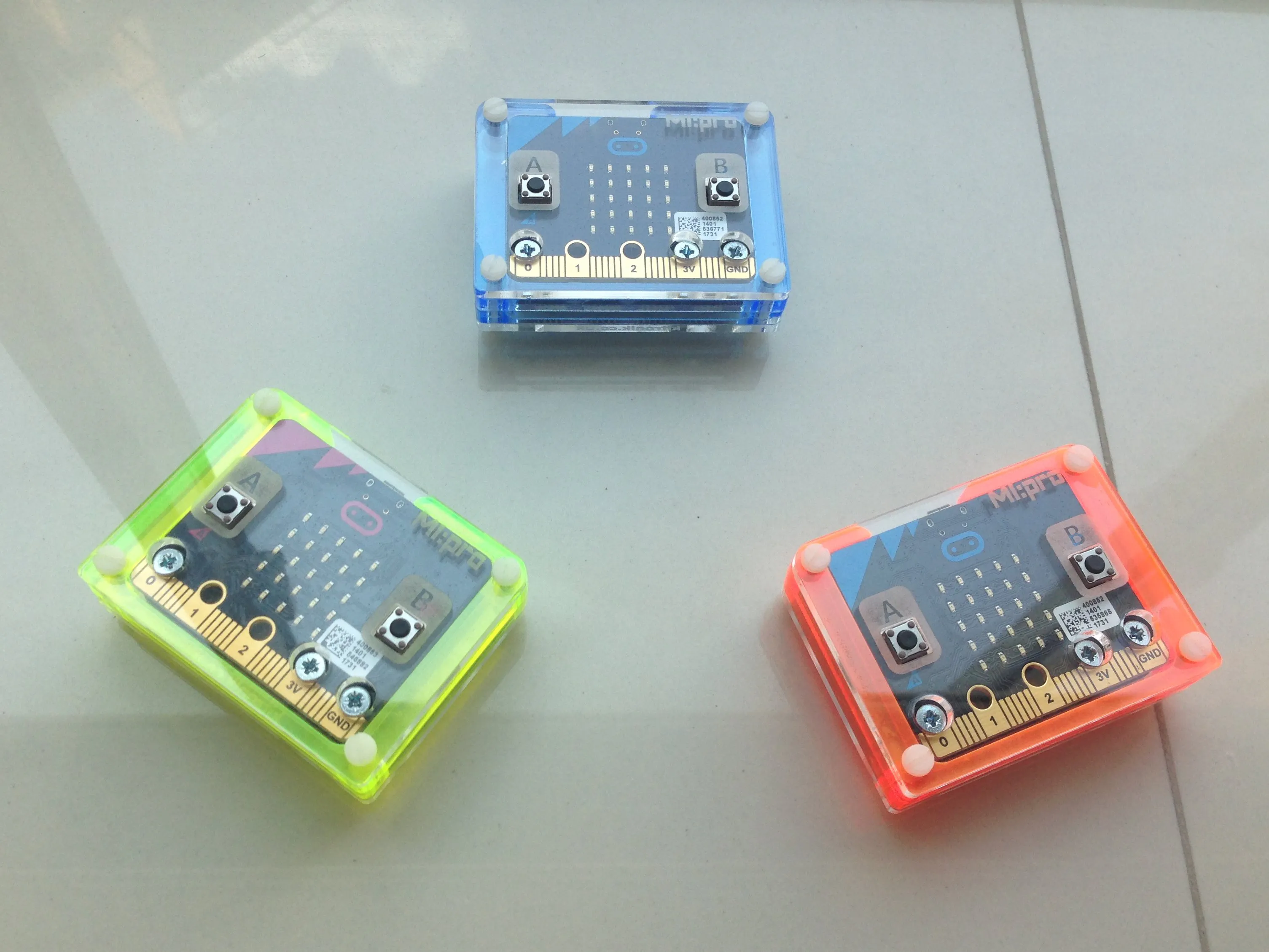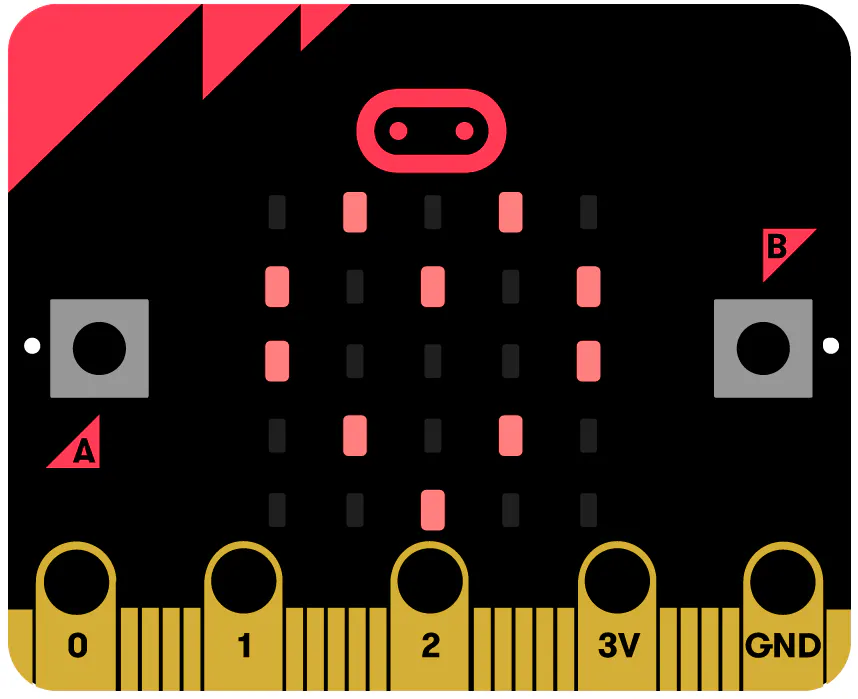Published

# Competitive Math Training Thru Microbits (Master Version)

A Master Microbit(Teacher) issues out math equations to Client Microbits(Students). Students then compete to be first w/ the correct answer.

IntermediateFull instructions provided1 hour1,747## Things used in this project

### Hardware componentsBBC micro:bit board
×1
 Kitronik MI:Power Board
×1
 Kitronik MI:pro Protector Case
×1

## Code

### A Microbit-Based Competitive Math Training Game (Master Version)

JavaScript
The Master program of a Microbit-based competitive math training game.
```let EqString = ""
let GotCorrect = 0
let CorrectID = 0
let Y = 0
let ShowAnsSw = 0
let X = 0
let PressButtA = 0
let Lvl = 0
let Eqn = 0
let ShowEqStr = 0
let serial2 = 0
input.onButtonPressed(Button.A, () => {
if (PressButtA == 0) {
if (Lvl == 0) {
X = Math.random(16)
Y = Math.random(16)
} else {
X = Math.random(41)
Y = Math.random(40)
}
X += 10
Y += 10
Eqn = X * 100
Eqn += Y
EqString = "" + X + "+" + Y
PressButtA = 1
}
ShowEqStr = 1
ShowAnsSw = 0
})
input.onButtonPressed(Button.B, () => {
if (PressButtA == 1) {
ShowEqStr = 0
ShowAnsSw = 1
}
})
if (name == "ANS") {
if (GotCorrect == 0) {
CorrectID = serial2
GotCorrect = 1
ShowAnsSw = 0
ShowEqStr = 0
for (let i = 0; i < 4; i++) {
basic.clearScreen()
basic.showIcon(IconNames.Yes)
}
music.beginMelody(music.builtInMelody(Melodies.PowerUp), MelodyOptions.Once)
}
}
}
}
})
input.onButtonPressed(Button.AB, () => {
for (let i = 0; i < 4; i++) {
basic.clearScreen()
}
Eqn = 0
GotCorrect = 0
X = 0
Y = 0
ShowEqStr = 0
PressButtA = 0
ShowAnsSw = 0
basic.clearScreen()
})
input.onGesture(Gesture.Shake, () => {
if (Lvl == 0) {
Lvl = 1
basic.clearScreen()
basic.showLeds(`
# . . # .
# . # # .
# . . # .
# . . # .
# # . # .
`)
basic.pause(5000)
} else {
Lvl = 0
basic.clearScreen()
basic.showLeds(`
# . . # .
# . # . #
# . # . #
# . # . #
# # . # .
`)
basic.pause(5000)
}
})
Eqn = 0
GotCorrect = 0
X = 0
Y = 0
ShowEqStr = 0
PressButtA = 0
ShowAnsSw = 0
EqString = ""
Lvl = 1
basic.forever(() => {
}
}
if (ShowEqStr == 1) {
basic.clearScreen()
basic.showString(EqString)
basic.pause(500)
}
if (ShowAnsSw == 1) {
basic.clearScreen()